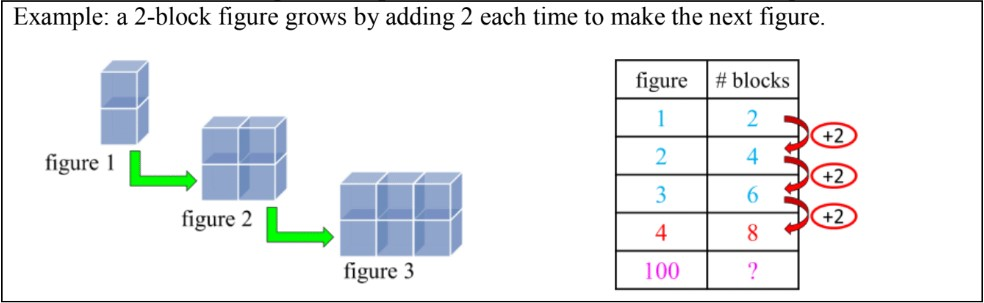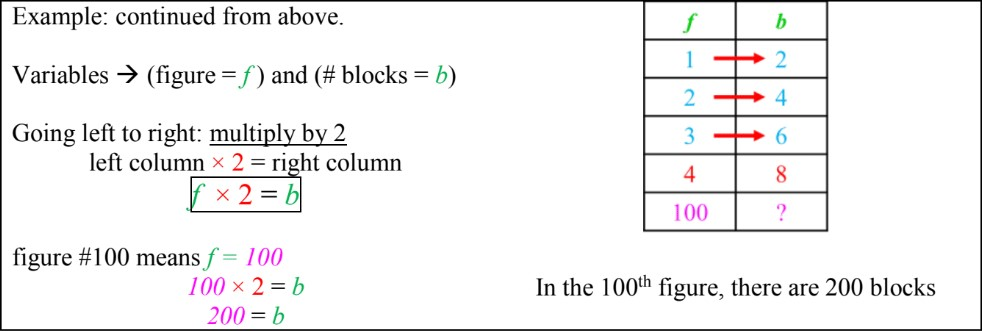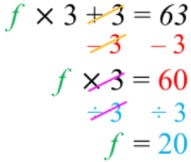# Introduction to solving patterns in T-tables and equations

### Introduction to solving patterns in T-tables and equations

#### Lessons

In this lesson, we will learn:

• How to determine and write one-step and two-step relationships in T-tables as equations with variables
• How to solve equations: isolating variables in two-step relationships (opposite operation to both sides)

Notes:

• Recall that T-tables keep track of patterns and allow calculation of subsequent terms• What is a fast way to find the 100th term? Adding by 2 each time is not efficient.
• T-table columns have a relationship between them. By writing this relationship as an equation, you can put in any number and find information about that term quickly.
• Ask: how do you go from the left column to the right column?
• Find one-step relationships through trial and error (if numbers are getting bigger it is + or ×; if numbers are getting smaller it is - or ÷)
• Assign variables to each column and use these variables in your equation• For more complicated patterns (two-step relationships) the equation will have two operations: the first step will be [× or ÷] and then the second step will be [+ or -]
• Similar to one-step relationships, they can be found through trial and error

• Isolating variables in two-step equations by “doing the opposite to both sides” in the backwards order of operations (solve by doing + or - first and then × or ÷ after)• Introduction
Introduction to Solving Patterns in T-tables and Equations:
a)
Finding one-step relationships between columns in a T-table

b)
Writing equations for T-table relationships (one-step)

c)
Writing equations for T-table relationships (two-step)

d)
How to isolate variables in two-step equations (solving algebra equations)

• 1.
T-tables and one-step equations
Write the equation for the relationship between the two variables. Follow the pattern to complete the T-table.
a)
variables $a$ and $b$
 a b 1 14 2 15 3 16 4 20

b)
variables $f$ and $g$
 f g 50 28 49 27 48 26 47 25

c)
variables $q$ and $r$
 q r 8 32 9 36 10 40 11 1

d)
variables $v$ and $w$
 v w 27 9 24 8 21 7 18 3

• 2.
T-tables and two-step equations
Write the equation for the relationship between the two variables. Follow the pattern to complete the T-table.
a)
variables $i$ and $j$
 i j 1 3 2 5 3 7 4 10

b)
variables $d$ and $e$
 d e 5 13 6 16 7 19 8 1

c)
variables $x$ and $y$
 x y 20 13 18 12 16 11 14 100

d)
variables $x$ and $y$
 x y 24 4 21 3 18 2 15 300

• 3.
T-tables and equations: word problem - 1
It costs $8 to rent a bicycle for the first hour. It costs$2 every hour after that.
a)
Fill out a T-table for the cost of the first five hours.
 hour cost

b)
Write an equation with variables for the relationship between hours ($h$) and the cost ($C$).

c)
How much would it cost to rent a bicycle for 24 hours?

d)
If someone paid a total of \$26, how many hours did they rent a bicycle for?

• 4.
T-tables and equations: word problem - 2
After the first month, seed A grew into a 2cm plant and continued to grow 5cm each month after that. Seed B grew to 6cm and continued to grow 3 cm each month after that.
a)
Fill out a T-table for the cost of the first three months for both seeds.
 month heightA
 month heightB

b)
Write an equation for each seed's growth, relating the number of months ($m$A, $m$B) and the height ($H$A, $H$B).

c)
After 1 year, what is the height difference between the two plants?

d)
When will each plant reach 42cm in height?